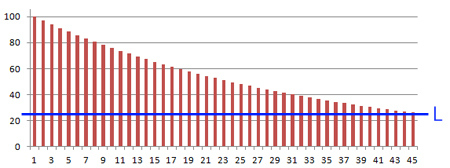# What is the current worth of your passive income?

With this calculation you can find out much the current worth is for a source of passive income. You can use this calculation to get an estimated sales price.

The calculation is based on the idea that a source of passive income is worth as much as it would profit until the source is deprecated, considering a decline over time (the decline in the graph).

In this calculation this is estimated by providing a lower limit where the profit is 'insignificant' (L in the graph). The accumulated income until in that happens is the same as the sum of the income reached until that time ('sum of the columns' in the graph).

You can also provide a rebate or margin (similar to raising, or lowering, the entire graph).#### What is broken?

Is there something wrong with the calculation What is the current worth of your passive income?? Is it a bug or has it gone completely offline? Please, let us know what is wrong! If the calculation did not give you the result you expected, please write which values you used and what you expected the calculation to do.

#### Bugs & Fixes

This calculation was last reviewed or fixed: 2011/09/29

Bug / Fix When Name Comment